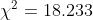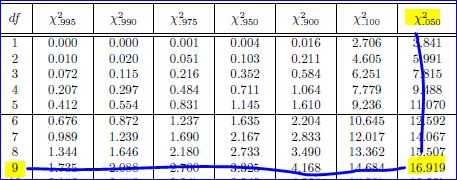##### Question

In: Statistics and Probability

# Conduct the hypothesis test and provide the test statistic and the critical​ value, and state the...

Conduct the hypothesis test and provide the test statistic and the critical​ value, and state the conclusion. A person purchased a slot machine and tested it by playing it 1,156 times. There are 10 different categories of​ outcomes, including no​ win, win​ jackpot, win with three​ bells, and so on. When testing the claim that the observed outcomes agree with the expected​ frequencies, the author obtained a test statistic of chi squared equals18.233. Use a 0.05 significance level to test the claim that the actual outcomes agree with the expected frequencies. Does the slot machine appear to be functioning as​ expected?

## Solutions

##### Expert Solution

Solution:

Given: A person purchased a slot machine and tested it by playing it 1,156 times. There are 10 different categories of​ outcomes, including no​ win, win​ jackpot, win with three​ bells, and so on.

Thus k = Number of categories = 10

The obtained test statistic of chi squared = 18.233

Level of significance = 0.05

We have to test the claim that the actual outcomes agree with the expected frequencies.

Hypothesis of study are:

H0: The actual outcomes agree with the expected frequencies

Vs

H1: The actual outcomes do not agree with the expected frequencies

Test statistic:Critical value:

df = k - 1 = 10 - 1 = 9

Level of significance = 0.05From Chi-square critical value table, we get:

Chi-square critical value = 16.919

Decision Rule: Reject H0, if Chi-square test statistic value > Chi-square critical value = 16.919, otherwise we fail to reject H0.

Since Chi-square test statistic value = 18.233 > Chi-square critical value = 16.919, we reject H0.

Conclusion:

Since we have rejected null hypothesis H0, there is not sufficient evidence to support the claim that the actual outcomes agree with the expected frequencies.

Thus the slot machine does not appear to be functioning as​ expected.

## Related Solutions

##### Conduct the hypothesis test and provide the test statistic and the critical? value, and state the...
Conduct the hypothesis test and provide the test statistic and the critical? value, and state the conclusion. A company claims that its packages of 100 candies are distributed with the following color? percentages: 13?% red, 22?%? orange, 16?%? yellow, 8?%? brown, 23?% blue, and 18?% green. Use the given sample data to test the claim that the color distribution is as claimed. Use a 0.10 significance level. candy count color number in package red 12 orange 25 yellow 8 brown...
##### Conduct the hypothesis test and provide the test? statistic, critical value and? P-value, and state the...
Conduct the hypothesis test and provide the test? statistic, critical value and? P-value, and state the conclusion. A person randomly selected 100100 checks and recorded the cents portions of those checks. The table below lists those cents portions categorized according to the indicated values. Use a 0.100.10 significance level to test the claim that the four categories are equally likely. The person expected that many checks for whole dollar amounts would result in a disproportionately high frequency for the first?...
##### Conduct the hypothesis test and provide the test​ statistic, critical value, and​ P-value, and state the...
Conduct the hypothesis test and provide the test​ statistic, critical value, and​ P-value, and state the conclusion. A person randomly selected 100 checks and recorded the cents portions of those checks. The table below lists those cents portions categorized according to the indicated values. Use a 0.10 significance level to test the claim that the four categories are equally likely. The person expected that many checks for whole dollar amounts would result in a disproportionately high frequency for the first​...
##### Conduct the hypothesis test and provide the test​ statistic, critical value and​ P-value, and state the...
Conduct the hypothesis test and provide the test​ statistic, critical value and​ P-value, and state the conclusion. A person drilled a hole in a die and filled it with a lead​ weight, then proceeded to roll it 200 times. Here are the observed frequencies for the outcomes of​ 1, 2,​ 3, 4,​ 5, and​ 6, respectively: 28​, 31​, 44​, 38​, 26​, 33. Use a 0.025 significance level to test the claim that the outcomes are not equally likely. Does it...
##### Conduct the hypothesis test and provide the test​ statistic, critical value and​ P-value, and state the...
Conduct the hypothesis test and provide the test​ statistic, critical value and​ P-value, and state the conclusion. A person drilled a hole in a die and filled it with a lead​ weight, then proceeded to roll it 200200 times. Here are the observed frequencies for the outcomes of​ 1, 2,​ 3, 4,​ 5, and​ 6, respectively: 2626​, 3030​, 4848​, 4141​, 2828​, 2727. Use a 0.010.01 significance level to test the claim that the outcomes are not equally likely. Does it...
##### Test the given claim. Identify the null​ hypothesis, alternative​ hypothesis, test​ statistic, P-value, and then state...
Test the given claim. Identify the null​ hypothesis, alternative​ hypothesis, test​ statistic, P-value, and then state the conclusion about the null​ hypothesis, as well as the final conclusion that addresses the original claim. Among 2083 passenger cars in a particular​ region, 230 had only rear license plates. Among 325 commercial​ trucks, 47 had only rear license plates. A reasonable hypothesis is that commercial trucks owners violate laws requiring front license plates at a higher rate than owners of passenger cars....
##### Test the given claim. Identify the null​ hypothesis, alternative​ hypothesis, test​ statistic, P-value, and then state...
Test the given claim. Identify the null​ hypothesis, alternative​ hypothesis, test​ statistic, P-value, and then state the conclusion about the null​ hypothesis, as well as the final conclusion that addresses the original claim. Among 2084 passenger cars in a particular​ region, 241 had only rear license plates. Among 349 commercial​ trucks, 53 had only rear license plates. A reasonable hypothesis is that commercial trucks owners violate laws requiring front license plates at a higher rate than owners of passenger cars....
##### Test the given claim. Identify the null hypothesis, alternative hypothesis, test statistic, P-value, and then state...
Test the given claim. Identify the null hypothesis, alternative hypothesis, test statistic, P-value, and then state the conclusion about the null hypothesis, as well as the final conclusion that addresses the original claim. Among 2173 passenger cars in a particular region, 235 had only rear license plates. Among 335335 commercial trucks, 5151 had only rear license plates. A reasonable hypothesis is that commercial trucks owners violate laws requiring front license plates at a higher rate than owners of passenger cars....
##### Test the given claim. Identify the null​ hypothesis, alternative​ hypothesis, test​ statistic, P-value, and then state...
Test the given claim. Identify the null​ hypothesis, alternative​ hypothesis, test​ statistic, P-value, and then state the conclusion about the null​ hypothesis, as well as the final conclusion that addresses the original claim. Among 2091 passenger cars in a particular​ region, 226 had only rear license plates. Among 375 commercial​ trucks, 56 had only rear license plates. A reasonable hypothesis is that commercial trucks owners violate laws requiring front license plates at a higher rate than owners of passenger cars....
##### Test the given claim. Identify the null​ hypothesis, alternative​ hypothesis, test​ statistic, P-value, and then state...
Test the given claim. Identify the null​ hypothesis, alternative​ hypothesis, test​ statistic, P-value, and then state the conclusion about the null​ hypothesis, as well as the final conclusion that addresses the original claim. Among 21952195 passenger cars in a particular​ region, 245245 had only rear license plates. Among 369369 commercial​ trucks, 5757 had only rear license plates. A reasonable hypothesis is that commercial trucks owners violate laws requiring front license plates at a higher rate than owners of passenger cars....